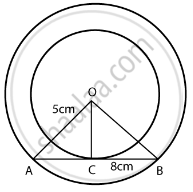# Out of the two concentric circles, the radius of the outer circle is 5 cm and the chord AC of length 8 cm is a tangent to the inner circle. Find the radius of the inner circle. - Mathematics

Short Note

Out of the two concentric circles, the radius of the outer circle is 5 cm and the chord AC of length 8 cm is a tangent to the inner circle. Find the radius of the inner circle.

#### SolutionFrom the figure,

Chord AB = 8 cm

OC is perpendicular to the chord AB

AC = CB = 4 cm

In right triangle OCA

OC2 + CA2 = OA2

OC2 = 5– 42 = 25 – 16 = 9

OC = 3 cm

Concept: Tangent to a Circle
Is there an error in this question or solution?

#### APPEARS IN

NCERT Mathematics Exemplar Class 10
Chapter 9 Circles
Exercise 9.3 | Q 1 | Page 107
RD Sharma Class 10 Maths
Chapter 8 Circles
Exercise 8.2 | Q 6 | Page 33
Share